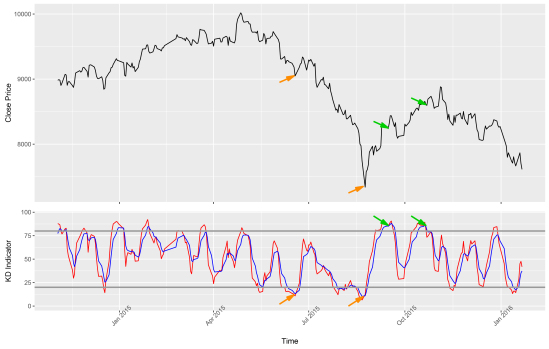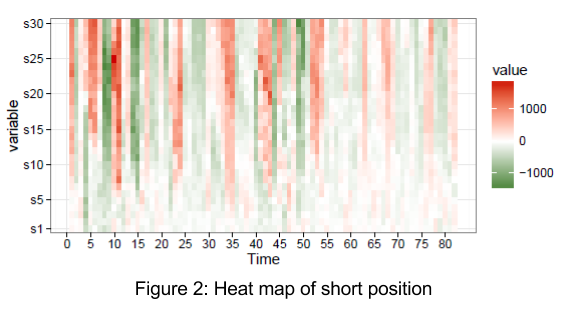策略無限

# 技術分析 — KD 指標入門指南

2019 年 1 月 8 日## 什麼是 KD 指標？

“ KD 指標” 又稱隨機指標（Stochastic Oscillator）並非一個數值，而是由 K 和 D 兩個值所組成，在時間軸上可以藉由兩個 K 值和 D 值走勢來判斷是否該進場或出場。其中一部分大家耳熟能詳的 “黃金交叉”、”死亡交叉” 也是來自這裡，藉由 KD 值交叉情形來判斷買進賣出的訊號。

• RSV 未成熟隨機值 （ Raw Stochastic Value ）：$$RSV = \frac{C_n-L_n}{H_n-L_n} \times 100\%$$
• K 值：$$K_n = \alpha\cdot RSV+(1-\alpha)\cdot K_{n-1}$$
• D 值：$$D_n = \alpha\cdot K_n+(1-\alpha)\cdot D_{n-1}$$

• $$n$$：經過的交易時間
• $$C_n$$ ：當日的收盤價
• $$H_n$$ ：過去 $$n$$ 日內的最高價
• $$L_n$$ ：過去 $$n$$ 日內的最低價

## 投資策略－黃金交叉、死亡交叉

• 黃金交叉: K<20, D<20, K 由下向上穿越 D 線
• 死亡交叉: K>80, D>80, K 由上向下穿越 D 線## 歷史報酬檢視從圖 2, 3 可以得到 3 個重要訊息:

1. 損益近幾年逐漸淡化，損益從圖 2 的第 55 筆（2010/6/20）訊息開始淡化，圖 3 的第 25 筆（2008/10/21）開始淡化，這表示在 2008 年以前使用 KD 指標做為參考依據比較容易有大賺大賠的情況發生，而在 2010 年後依據 KD 指標作為投資策略的損益較小。
2. 損益隨著持有的時間增加，不難想像越長的持有天期損益會更加劇烈，持有日期 5 天以內的顏色很淡，表示損益較遠天期的持有損益小，從另一個角度想或許可以透過越長的持有天期來調整實際損益。
3. 從熱圖發現由 KD 指標作為投資策略的損益，會有群聚效應，在某個時間點，若賺錢的話會一直賺錢，賠錢的話則會一直賠錢，像是有週期性的反覆出現，圖 2 的周期較短，圖 3 的周期較長。

## 結語

1. 因為 KD 指標公式的特性，只會考慮目前收盤價位於 n 天內相對高點或低點，如果未來出現長期趨勢的向下或向上波段，只參考 KD 指標會容易出現失誤。
2. 依據 80-20 制訂的策略進行投資，近期的投資較容易出現小賺小賠的情況。

【延伸閱讀】策略無限